# Section 21.6. Handcoded Parsers

### 21.6. Handcoded Parsers

Since Python is a general-purpose programming language, it's also reasonable to consider writing a handcoded parser. For instance, recursive descent parsing is a fairly well-known technique for analyzing language-based information. Since Python is a very high-level language, writing the parser itself is usually easier than it would be in a traditional language such as C or C++.

To illustrate, this section develops a custom parser for a simple grammar: it parses and evaluates arithmetic expression strings. This example also demonstrates the utility of Python as a general-purpose programming language. Although Python is often used as a frontend or rapid development language, it's also useful for the kinds of things we'd normally write in a systems development language such as C or C++.

#### 21.6.1. The Expression Grammar

The grammar that our parser will recognize can be described as follows:

` goal -> <expr> END                       [number, variable, ( ] goal -> <assign> END                     [set] assign -> 'set' <variable> <expr>        [set] expr -> <factor> <expr-tail>             [number, variable, ( ] expr-tail -> ^                           [END, ) ] expr-tail -> '+' <factor> <expr-tail>    [+] expr-tail -> '-' <factor> <expr-tail>    [-] factor -> <term> <factor-tail>           [number, variable, ( ] factor-tail -> ^                         [+, -, END, ) ] factor-tail -> '*' <term> <factor-tail>  [*] factor-tail -> '/' <term> <factor-tail>  [/] term -> <number>                         [number] term -> <variable>                       [variable] term -> '(' <expr> ')'                   [(] tokens: (, ), num, var, -, +, /, *, set, end `

This is a fairly typical grammar for a simple expression language, and it allows for arbitrary expression nesting (some example expressions appear at the end of the testparser module listing in Example 21-11). Strings to be parsed are either an expression or an assignment to a variable name (set). Expressions involve numbers, variables, and the operators +, -, *, and /. Because factor is nested in expr in the grammar, * and / have higher precedence (i.e., they bind tighter) than + and -. Expressions can be enclosed in parentheses to override precedence, and all operators are left associativethat is, they group on the left (e.g., 1-2-3 is treated the same as (1-2)-3).

Tokens are just the most primitive components of the expression language. Each grammar rule earlier is followed in square brackets by a list of tokens used to select it. In recursive descent parsing, we determine the set of tokens that can possibly start a rule's substring, and we use that information to predict which rule will work ahead of time. For rules that iterate (the -tail rules), we use the set of possibly following tokens to know when to stop. Typically, tokens are recognized by a string processor (a scanner), and a higher-level processor (a parser) uses the token stream to predict and step through grammar rules and substrings.

#### 21.6.2. The Parser's Code

The system is structured as two modules, holding two classes:

• The scanner handles low-level character-by-character analysis.

• The parser embeds a scanner and handles higher-level grammar analysis.

The parser is also responsible for computing the expression's value and testing the system. In this version, the parser evaluates the expression while it is being parsed. To use the system, we create a parser with an input string and call its parse method. We can also call parse again later with a new expression string.

There's a deliberate division of labor here. The scanner extracts tokens from the string, but it knows nothing about the grammar. The parser handles the grammar, but it is naive about the string itself. This modular structure keeps the code relatively simple. And it's another example of the object-oriented programming (OOP) composition relationship at work: parsers embed and delegate to scanners.

The module in Example 21-9 implements the lexical analysis taskdetecting the expression's basic tokens by scanning the text string left to right on demand. Notice that this is all straightforward logic; such analysis can sometimes be performed with regular expressions instead (described earlier), but the pattern needed to detect and extract tokens in this example would be too complex and fragile for my tastes. If your tastes vary, try recoding this module with re.

##### Example 21-9. PP3E\Lang\Parser\scanner.py

 ` #################################################### # the scanner (lexical analyser) #################################################### import string class SyntaxError(Exception): pass # local errors class LexicalError(Exception): pass # used to be strings class Scanner: def _ _init_ _(self, text): self.next = 0 self.text = text + '\0' def newtext(self, text): Scanner._ _init_ _(self, text) def showerror(self): print '=> ', self.text print '=> ', (' ' * self.start) + '^' def match(self, token): if self.token != token: raise SyntaxError, [token] else: value = self.value if self.token != '\0': self.scan( ) # next token/value return value # return prior value def scan(self): self.value = None ix = self.next while self.text[ix] in string.whitespace: ix = ix+1 self.start = ix if self.text[ix] in ['(', ')', '-', '+', '/', '*', '\0']: self.token = self.text[ix] ix = ix+1 elif self.text[ix] in string.digits: str = '' while self.text[ix] in string.digits: str = str + self.text[ix] ix = ix+1 if self.text[ix] == '.': str = str + '.' ix = ix+1 while self.text[ix] in string.digits: str = str + self.text[ix] ix = ix+1 self.token = 'num' self.value = float(str) else: self.token = 'num' self.value = long(str) elif self.text[ix] in string.letters: str = '' while self.text[ix] in (string.digits + string.letters): str = str + self.text[ix] ix = ix+1 if str.lower( ) == 'set': self.token = 'set' else: self.token = 'var' self.value = str else: raise LexicalError self.next = ix `

The parser module's class creates and embeds a scanner for its lexical chores and handles interpretation of the expression grammar's rules and evaluation of the expression's result, as shown in Example 21-10.

##### Example 21-10. PP3E\Lang\Parser\parser1.py

 ` ######################################################## # the parser (syntax analyser, evaluates during parse) ######################################################## class UndefinedError(Exception): pass from scanner import Scanner, LexicalError, SyntaxError class Parser: def _ _init_ _(self, text=''): self.lex = Scanner(text) # embed a scanner self.vars = {'pi':3.14159} # add a variable def parse(self, *text): if text: # main entry-point self.lex.newtext(text) # reuse this parser? try: self.lex.scan( ) # get first token self.Goal( ) # parse a sentence except SyntaxError: print 'Syntax Error at column:', self.lex.start self.lex.showerror( ) except LexicalError: print 'Lexical Error at column:', self.lex.start self.lex.showerror( ) except UndefinedError, name: print "'%s' is undefined at column:" % name, self.lex.start self.lex.showerror( ) def Goal(self): if self.lex.token in ['num', 'var', '(']: val = self.Expr( ) self.lex.match('\0') # expression? print val elif self.lex.token == 'set': # set command? self.Assign( ) self.lex.match('\0') else: raise SyntaxError def Assign(self): self.lex.match('set') var = self.lex.match('var') val = self.Expr( ) self.vars[var] = val # assign name in dict def Expr(self): left = self.Factor( ) while 1: if self.lex.token in ['\0', ')']: return left elif self.lex.token == '+': self.lex.scan( ) left = left + self.Factor( ) elif self.lex.token == '-': self.lex.scan( ) left = left - self.Factor( ) else: raise SyntaxError def Factor(self): left = self.Term( ) while 1: if self.lex.token in ['+', '-', '\0', ')']: return left elif self.lex.token == '*': self.lex.scan( ) left = left * self.Term( ) elif self.lex.token == '/': self.lex.scan( ) left = left / self.Term( ) else: raise SyntaxError def Term(self): if self.lex.token == 'num': val = self.lex.match('num') # numbers return val elif self.lex.token == 'var': if self.vars.has_key(self.lex.value): val = self.vars[self.lex.value] # look up name's value self.lex.scan( ) return val else: raise UndefinedError, self.lex.value elif self.lex.token == '(': self.lex.scan( ) val = self.Expr( ) # sub-expression self.lex.match(')') return val else: raise SyntaxError if _ _name_ _ == '_ _main_ _': import testparser # self-test code testparser.test(Parser, 'parser1') # test local Parser `

If you study this code closely, you'll notice that the parser keeps a dictionary (self.vars) to manage variable names: they're stored in the dictionary on a set command and are fetched from it when they appear in an expression. Tokens are represented as strings, with an optional associated value (a numeric value for numbers and a string for variable names).

The parser uses iteration (while loops) rather than recursion for the expr-tail and factor-tail rules. Other than this optimization, the rules of the grammar map directly onto parser methods: tokens become calls to the scanner, and nested rule references become calls to other methods.

When the file parser1.py is run as a top-level program, its self-test code is executed, which in turn simply runs a canned test in the module shown in Example 21-11. Note that all integer math uses Python long integers (unlimited precision integers) because the scanner converts numbers to strings with long. Also notice that mixed integer/floating-point operations cast up to floating point since Python operators are used to do the actual calculations.

##### Example 21-11. PP3E\Lang\Parser\testparser.py

 ` #################################################### # parser test code #################################################### def test(ParserClass, msg): print msg, ParserClass x = ParserClass('4 / 2 + 3') # allow different Parser's x.parse( ) x.parse('3 + 4 / 2') # like eval('3 + 4 / 2')... x.parse('(3 + 4) / 2') x.parse('4 / (2 + 3)') x.parse('4.0 / (2 + 3)') x.parse('4 / (2.0 + 3)') x.parse('4.0 / 2 * 3') x.parse('(4.0 / 2) * 3') x.parse('4.0 / (2 * 3)') x.parse('(((3))) + 1') y = ParserClass( ) y.parse('set a 4 / 2 + 1') y.parse('a * 3') y.parse('set b 12 / a') y.parse('b') z = ParserClass( ) z.parse('set a 99') z.parse('set a a + 1') z.parse('a') z = ParserClass( ) z.parse('pi') z.parse('2 * pi') z.parse('1.234 + 2.1') def interact(ParserClass): # command-line entry print ParserClass x = ParserClass( ) while 1: cmd = raw_input('Enter=> ') if cmd == 'stop': break x.parse(cmd) `

Correlate the following results to print statements in the self-test module:

` C:\...\PP3E\Lang\Parser>python parser1.py parser1 _ _main_ _.Parser 5 5 3 0 0.8 0.8 6.0 6.0 0.666666666667 4 9 4 100 3.14159 6.28318 3.334 `

The integer results here are really long integers; in the past they printed with a trailing L (e.g., 5L), but they no longer do in recent Python releases because normal integers are automatically converted to long integers as needed (in fact, there may be no distinction between the two types at all in a future Python release). Change the Goal method to print repr(val) if you still want to see the Lrepr prints objects as code.

As usual, we can also test and use the system interactively:

` % python >>> import parser1 >>> x = parser1.Parser( ) >>> x.parse('1 + 2') 3 `

Error cases are trapped and reported:

` >>> x.parse('1 + a') 'a' is undefined at column: 4 =>  1 + a =>      ^ >>> x.parse('1+a+2') 'a' is undefined at column: 2 =>  1+a+2 =>    ^ >>> x.parse('1 * 2 \$') Lexical Error at column: 6 =>  1 * 2 \$ =>        ^ >>> x.parse('1 * - 1') Syntax Error at column: 4 =>  1 * - 1 =>      ^ >>> x.parse('1 * (9') Syntax Error at column: 6 =>  1 * (9 =>        ^ `

Pathologically big numbers are handled well, because Python's built-in objects and operators are used along the way:

` >>> x.parse('888888888888888888888888888888888888888888888.9999999') 8.88888888889e+044 >>> x.parse('99999999999999999999999999999999999999999 + 2') 100000000000000000000000000000000000000001 >>> x.parse('999999999999999999999999999999.88888888888 + 1.1') 1e+030 `

In addition, there is an interactive loop interface in the testparser module if you want to use the parser as a simple command-line calculator (or if you get tired of typing parser method calls). Pass the Parser class, so testparser can make one of its own:

` >>> import testparser >>> testparser.interact(parser1.Parser) Enter=> 4 * 3 + 5 17 Enter=> 5 + 4 * 3 17 Enter=> (5 + 4) * 3 27 Enter=> set a 99 Enter=> set b 66 Enter=> a + b 165 Enter=> # + 1 Lexical Error at column: 0 =>  # + 1 =>  ^ Enter=> a * b + c 'c' is undefined at column: 8 =>  a * b + c =>          ^ Enter=> a * b * + c Syntax Error at column: 8 =>  a * b * + c =>          ^ Enter=> a 99 Enter=> a * a * a 970299 Enter=> stop >>> `

## Lesson 3: Divide and Conquer

As the parser system demonstrates, modular program design is almost always a major win. By using Python's program structuring tools (functions, modules, classes, and so on), big tasks can be broken down into small, manageable parts that can be coded and tested independently.

For instance, the scanner can be tested without the parser by making an instance with an input string and calling its scan or match methods repeatedly. We can even test it like this interactively, from Python's command line. By separating programs into logical components, they become easier to understand and modify. Imagine what the parser would look like if the scanner's logic was embedded rather than called.

#### 21.6.3. Adding a Parse Tree Interpreter

One weakness in the parser1 program is that it embeds expression evaluation logic in the parsing logic: the result is computed while the string is being parsed. This makes evaluation quick, but it can also make it difficult to modify the code, especially in larger systems. To simplify, we could restructure the program to keep expression parsing and evaluation separate. Instead of evaluating the string, the parser can build up an intermediate representation of it that can be evaluated later. As an added incentive, building the representation separately makes it available to other analysis tools (e.g., optimizers, viewers, and so on)they can be run as separate passes over the tree.

Example 21-12 shows a variant of parser1 that implements this idea. The parser analyzes the string and builds up a parse treethat is, a tree of class instances that represents the expression and that may be evaluated in a separate step. The parse tree is built from classes that "know" how to evaluate themselves: to compute the expression, we just ask the tree to evaluate itself. Root nodes in the tree ask their children to evaluate themselves, and then combine the results by applying a single operator. In effect, evaluation in this version is simply a recursive traversal of a tree of embedded class instances constructed by the parser.

##### Example 21-12. PP3E\Lang\Parser\parser2.py

 ` TraceDefault = False class UndefinedError(Exception): pass from scanner import Scanner, SyntaxError, LexicalError #################################################### # the interpreter (a smart objects tree) #################################################### class TreeNode: def validate(self, dict): # default error check pass def apply(self, dict): # default evaluator pass def trace(self, level): # default unparser print '.'*level + '' # ROOTS class BinaryNode(TreeNode): def _ _init_ _(self, left, right): # inherited methods self.left, self.right = left, right # left/right branches def validate(self, dict): self.left.validate(dict) # recurse down branches self.right.validate(dict) def trace(self, level): print '.'*level + '[' + self.label + ']' self.left.trace(level+3) self.right.trace(level+3) class TimesNode(BinaryNode): label = '*' def apply(self, dict): return self.left.apply(dict) * self.right.apply(dict) class DivideNode(BinaryNode): label = '/' def apply(self, dict): return self.left.apply(dict) / self.right.apply(dict) class PlusNode(BinaryNode): label = '+' def apply(self, dict): return self.left.apply(dict) + self.right.apply(dict) class MinusNode(BinaryNode): label = '-' def apply(self, dict): return self.left.apply(dict) - self.right.apply(dict) # LEAVES class NumNode(TreeNode): def _ _init_ _(self, num): self.num = num # already numeric def apply(self, dict): # use default validate return self.num def trace(self, level): print '.'*level + repr(self.num) # as code, was 'self.num' class VarNode(TreeNode): def _ _init_ _(self, text, start): self.name = text # variable name self.column = start # column for errors def validate(self, dict): if not dict.has_key(self.name): raise UndefinedError, (self.name, self.column) def apply(self, dict): return dict[self.name] # validate before apply def assign(self, value, dict): dict[self.name] = value # local extension def trace(self, level): print '.'*level + self.name # COMPOSITES class AssignNode(TreeNode): def _ _init_ _(self, var, val): self.var, self.val = var, val def validate(self, dict): self.val.validate(dict) # don't validate var def apply(self, dict): self.var.assign( self.val.apply(dict), dict ) def trace(self, level): print '.'*level + 'set ' self.var.trace(level + 3) self.val.trace(level + 3) #################################################### # the parser (syntax analyser, tree builder) #################################################### class Parser: def _ _init_ _(self, text=''): self.lex = Scanner(text) # make a scanner self.vars = {'pi':3.14159} # add constants self.traceme = TraceDefault def parse(self, *text): # external interface if text: self.lex.newtext(text) # reuse with new text tree = self.analyse( ) # parse string if tree: if self.traceme: # dump parse-tree? print; tree.trace(0) if self.errorCheck(tree): # check names self.interpret(tree) # evaluate tree def analyse(self): try: self.lex.scan( ) # get first token return self.Goal( ) # build a parse-tree except SyntaxError: print 'Syntax Error at column:', self.lex.start self.lex.showerror( ) except LexicalError: print 'Lexical Error at column:', self.lex.start self.lex.showerror( ) def errorCheck(self, tree): try: tree.validate(self.vars) # error checker return 'ok' except UndefinedError, instance: # args is a tuple varinfo = instance.args # instance is a sequence print "'%s' is undefined at column: %d" % varinfo self.lex.start = varinfo self.lex.showerror( ) # returns None def interpret(self, tree): result = tree.apply(self.vars) # tree evals itself if result != None: # ignore 'set' result print result def Goal(self): if self.lex.token in ['num', 'var', '(']: tree = self.Expr( ) self.lex.match('\0') return tree elif self.lex.token == 'set': tree = self.Assign( ) self.lex.match('\0') return tree else: raise SyntaxError def Assign(self): self.lex.match('set') vartree = VarNode(self.lex.value, self.lex.start) self.lex.match('var') valtree = self.Expr( ) return AssignNode(vartree, valtree) # two subtrees def Expr(self): left = self.Factor( ) # left subtree while 1: if self.lex.token in ['\0', ')']: return left elif self.lex.token == '+': self.lex.scan( ) left = PlusNode(left, self.Factor( )) # add root-node elif self.lex.token == '-': self.lex.scan( ) left = MinusNode(left, self.Factor( )) # grows up/right else: raise SyntaxError def Factor(self): left = self.Term( ) while 1: if self.lex.token in ['+', '-', '\0', ')']: return left elif self.lex.token == '*': self.lex.scan( ) left = TimesNode(left, self.Term( )) elif self.lex.token == '/': self.lex.scan( ) left = DivideNode(left, self.Term( )) else: raise SyntaxError def Term(self): if self.lex.token == 'num': leaf = NumNode(self.lex.match('num')) return leaf elif self.lex.token == 'var': leaf = VarNode(self.lex.value, self.lex.start) self.lex.scan( ) return leaf elif self.lex.token == '(': self.lex.scan( ) tree = self.Expr( ) self.lex.match(')') return tree else: raise SyntaxError #################################################### # self-test code: use my parser, parser1's tester #################################################### if _ _name_ _ == '_ _main_ _': import testparser testparser.test(Parser, 'parser2') # run with Parser class here `

When parser2 is run as a top-level program, we get the same test code output as for parser1. In fact, it reuses the same test code: both parsers pass in their parser class object to testparser.test. And since classes are objects, we can also pass this version of the parser to testparser's interactive loop: testparser.interact(parser2.Parser). The new parser's external behavior is identical to that of the original.

Notice the way we handle undefined name exceptions in errorCheck. This exception is a class instance now, not a string as in the prior edition (string exceptions are now deprecated). When exceptions derived from the built-in Exception class are used as sequences, they return the arguments passed to the exception constructor call. This doesn't quite work for string formatting, though, because it expects a real tuplewe have to call tuple( ) manually on the instance, extract the arguments with its args attribute, or write our own custom constructor to handle the state information.

Also notice that the new parser reuses the same scanner module as well. To catch errors raised by the scanner, it also imports the specific strings that identify the scanner's exceptions. Both the scanner and the parser can raise exceptions on errors (lexical errors, syntax errors, and undefined name errors). They're caught at the top level of the parser, and they end the current parse. There's no need to set and check status flags to terminate the recursion. Since math is done using long integers, floating-point numbers, and Python's operators, there's usually no need to trap numeric overflow or underflow errors. But as is, the parser doesn't handle errors such as division by zerosuch errors make the parser system exit with a Python stack dump. Uncovering the cause and fix for this is left as an exercise.

#### 21.6.4. Parse Tree Structure

In fact, the only real difference with this latest parser is that it builds and uses trees to evaluate an expression internally instead of evaluating as it parses. The intermediate representation of an expression is a tree of class instances, whose shape reflects the order of operator evaluation. This parser also has logic to print an indented listing of the constructed parse tree if the TRaceme attribute is set to TRue (or 1). Integers print with a trailing L here because the trace logic displays them with repr, indentation gives the nesting of subtrees, and binary operators list left subtrees first. For example:

` % python >>> import parser2 >>> p = parser2.Parser( ) >>> p.traceme = 1 >>> p.parse('5 + 4 * 2') [+] ...5L ...[*] ......4L ......2L 13L `

When this tree is evaluated, the apply method recursively evaluates subtrees and applies root operators to their results. Here, * is evaluated before +, since it's lower in the tree. The Factor method consumes the * substring before returning a right subtree to Expr:

` >>> p.parse('5 * 4 - 2') [-] ...[*] ......5L ......4L ...2L 18L `

In this example, * is evaluated before -. The Factor method loops through a substring of * and / expressions before returning the resulting left subtree to Expr:

` >>> p.parse('1 + 3 * (2 * 3 + 4)') [+] ...1L ...[*] ......3L ......[+] .........[*] ............2L ............3L .........4L 31L `

Trees are made of nested class instances. From an OOP perspective, it's another way to use composition. Since tree nodes are just class instances, this tree could be created and evaluated manually too:

` PlusNode( NumNode(1),           TimesNode( NumNode(3),                      PlusNode( TimesNode(NumNode(2), NumNode(3)),                                NumNode(4) ))).apply({}) `

But we might as well let the parser build it for us (Python is not that much like Lisp, despite what you may have heard).

#### 21.6.5. Exploring Parse Trees with PyTree

But waitthere is a better way to explore parse tree structures. Figure 21-1 shows the parse tree generated for the string 1 + 3 * (2 * 3 + 4), displayed in PyTree, the tree visualization GUI presented at the end of Chapter 20. This works only because the parser2 module builds the parse tree explicitly (parser1 evaluates during a parse instead), and because PyTree's code is generic and reusable.

##### Figure 21-1. Parse tree built for 1 + 3 * (2 * 3 + 4)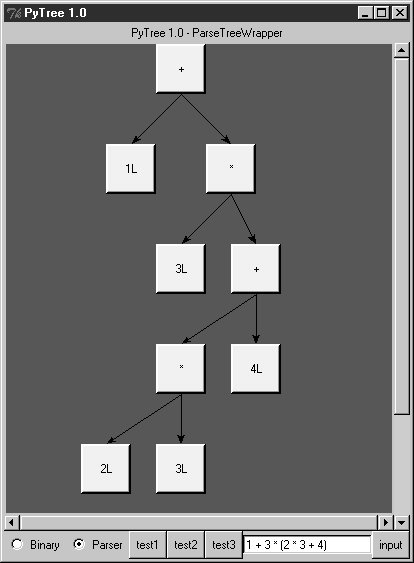If you read the last chapter, you'll recall that PyTree can draw most any tree data structure, but it is preconfigured to handle binary search trees and the parse trees we're studying in this chapter. You might also remember that clicking on nodes in a displayed parse tree evaluates the subtree rooted there. Figure 21-2 shows the pop up generated after clicking the tree's root node (you get different results if you click other parts of the tree because smaller subtrees are evaluated).

##### Figure 21-2. Clicking the root node to evaluate a tree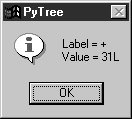PyTree makes it easy to learn about and experiment with the parser. To determine the tree shape produced for a given expression, start PyTree, click on its Parser radio button, type the expression in the input field at the bottom, and press "input" (or your Enter key). The parser class is run to generate a tree from your input, and the GUI displays the result. For instance, Figure 21-3 sketches the parse tree generated if we remove the parentheses from the first expression in the input field. The root node evaluates to 23 this time, due to the different shape's evaluation order.

##### Figure 21-3. Parse tree for 1 + 3 * 2 * 3 + 4, result=23To generate a shape that is even more different, try introducing more parentheses to the expression and hitting the Enter key again. Figure 21-4 shows a much flatter tree structure produced by adding a few parentheses to override operator precedence. Because these parentheses change the tree shape, they also change the expression's overall result again. Figure 21-5 shows the resulting pop up after clicking the root node in this display.

##### Figure 21-4. Parse tree built for (1 + 3) * (2 * (3 + 4))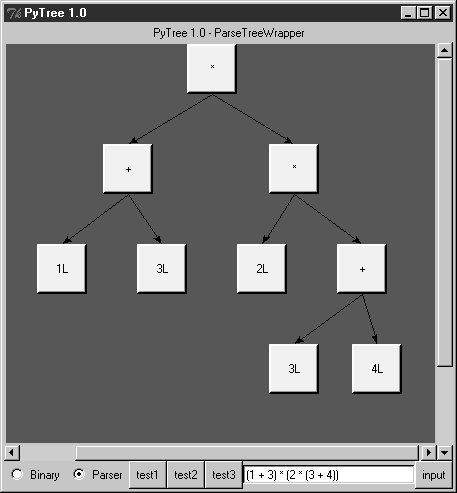##### Figure 21-5. Clicking and evaluating the root node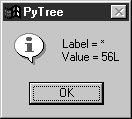Depending on the operators used within an expression, some very differently shaped trees yield the same result when evaluated. For instance, Figure 21-6 shows a more left-heavy tree generated from a different expression string that evaluates to 56 nevertheless.

##### Figure 21-6. Parse tree for (1 + 3) * 2 * (3 + 4), result=56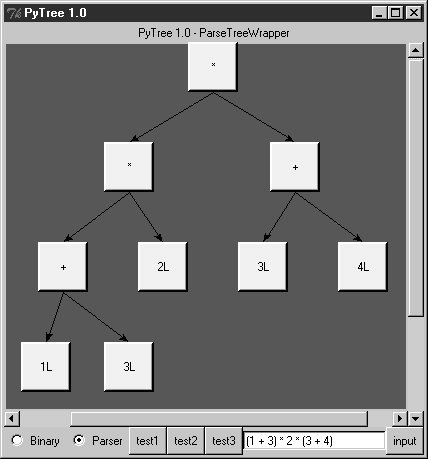Finally, Figure 21-7 shows a parsed assignment statement; clicking the set root assigns the variable spam, and clicking the node spam then evaluates to -4. If you find the parser puzzling, try running PyTree like this on your computer to get a better feel for the parsing process. (I'd like to show more example trees, but I ran out of page real estate at this point in the book.)

##### Figure 21-7. Assignment, left-grouping: "set spam 1 - 2 - 3"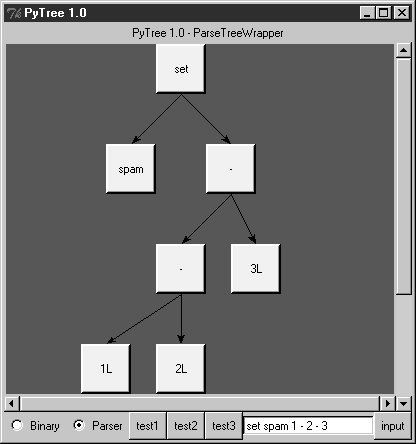#### 21.6.6. Parsers Versus Python

The handcoded parser programs shown earlier illustrate some interesting concepts and underscore the power of Python for general-purpose programming. Depending on your job description, they may also be typical of the sort of thing you'd write regularly in a traditional language such as C. Parsers are an important component in a wide variety of applications, but in some cases, they're not as necessary as you might think. Let me explain why.

So far, we started with an expression parser and added a parse tree interpreter to make the code easier to modify. As is, the parser works, but it may be slow compared to a C implementation. If the parser is used frequently, we could speed it up by moving parts to C extension modules. For instance, the scanner might be moved to C initially, since it's often called from the parser. Ultimately, we might add components to the grammar that allow expressions to access application-specific variables and functions.

All of these steps constitute good engineering. But depending on your application, this approach may not be the best one in Python. Often the easiest way to evaluate input expressions in Python is to let Python do it, by calling the eval built-in function. In fact, we can usually replace the entire expression evaluation program with one function call. The next example will demonstrate how this is done.

More important, the next section underscores a core idea behind the language: if you already have an extensible, embeddable, high-level language system, why invent another? Python itself can often satisfy language-based component needs.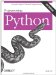Programming Python
ISBN: 0596009259
EAN: 2147483647
Year: 2004
Pages: 270
Authors: Mark Lutz

Similar book on Amazon